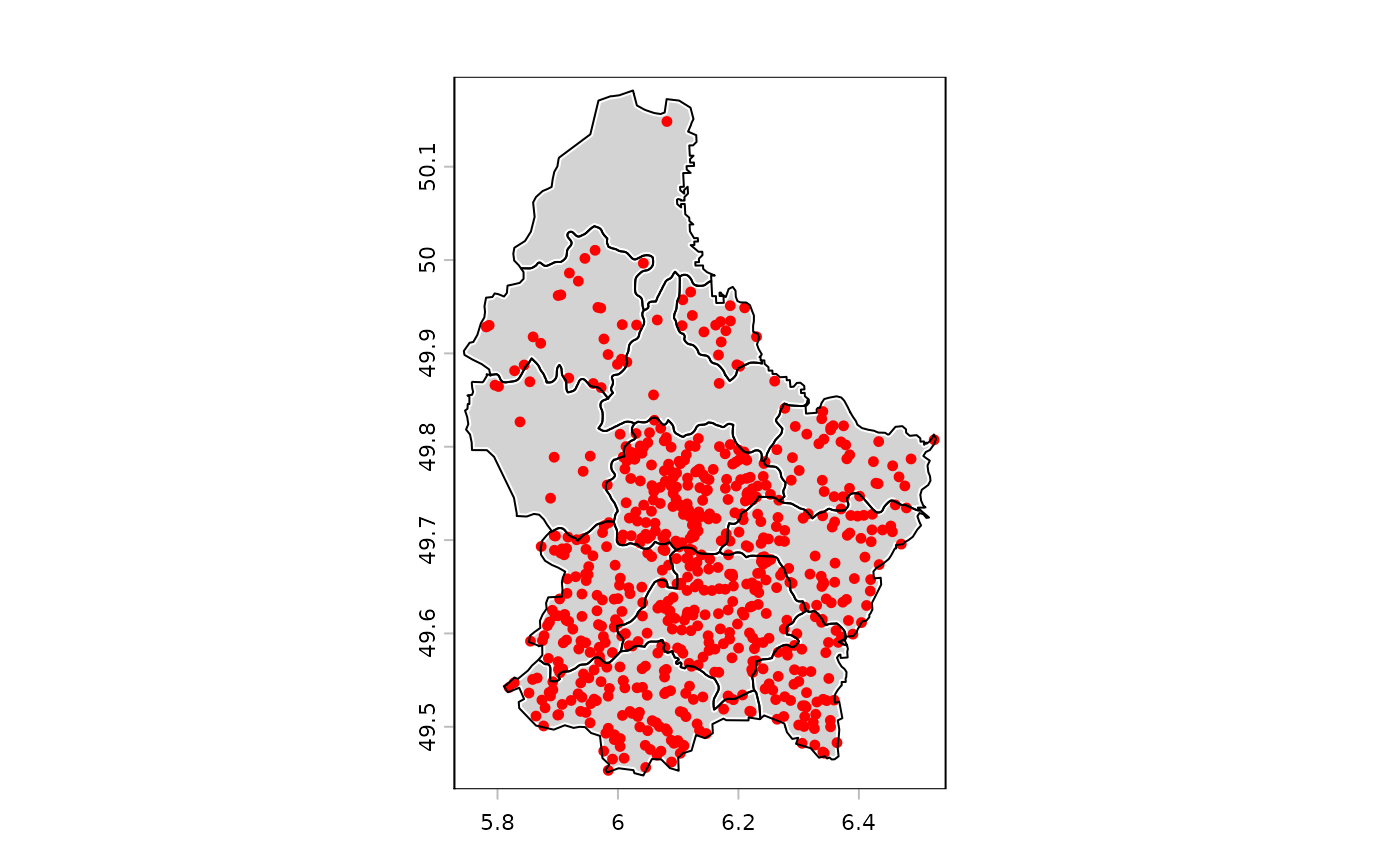Create the dots for a dot-density map and add these to the current map. Dot-density maps are made to display count data. For example of population counts, where each dot represents n persons. The dots are returned as a SpatVector. It there is an active graphics device, the dots are added to it with points.

# S4 method for SpatVector
dots(x, field, size,  ...)

## Arguments

x

SpatVector

field

character of numeric indicating field name. Or numeric vector of the same length as x

size

positive number indicating the number of cases associated with each dot

...

graphical arguments passed to points

## Value

SpatVector (invisibly)

plot, cartogram, points

## Examples

f <- system.file("ex/lux.shp", package="terra")
v <- vect(f)
v\$population <- 1000*(1:12)^2
plot(v, lwd=3, col="light gray", border="white")
d <- dots(v, "population", 1000, col="red", cex=.75)
lines(v)d
#>  class       : SpatVector
#>  geometry    : points
#>  dimensions  : 650, 7  (geometries, attributes)
#>  extent      : 5.774251, 6.515615, 49.45477, 50.03893  (xmin, xmax, ymin, ymax)
#>  coord. ref. : lon/lat WGS 84 (EPSG:4326)
#>  names       :  ID_1   NAME_1  ID_2   NAME_2  AREA   POP population
#>  type        : <num>    <chr> <num>    <chr> <num> <int>      <num>
#>  values      :     1 Diekirch     1 Clervaux   312 18081       1000
#>                    1 Diekirch     2 Diekirch   218 32543       4000
#>                    1 Diekirch     2 Diekirch   218 32543       4000